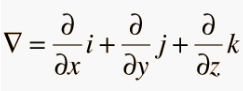# Del Operator (Nabla operator)

The Del Operator (also called the Nabla operator or the vector differential operator) is a mathematical operator (actually a collection of partial derivative operators) commonly used in vector calculus to find higher dimensional derivatives. An “operator” is similar to a function (Green, 1998).

Although it’s defined as a vector, it doesn’t have magnitude and so isn’t a “true” vector.By itself, it doesn’t actually have any meaning at all (much like a lonely multiplication or addition symbol). It only has a meaning when combined with a scalar function or vector function.

## Del Operator Uses

The del operator is used in various vector calculus operations. Depending on what expression the operator appears in, it may denote gradient of a scalar field, divergence of a vector field, curl of a vector field, or the Laplacian operator:

• Divergence (the operator equivalent of a scalar product of two vectors): ∇ x E
• Curl (the vector product equivalent of divergence): ∇ · E
• Laplacian: ∇ · ∇f = ∇2f

The del operator can be applied to a scalar function or a vector function. ∇ · F is a scalar function, and ∇ x F is a vector function.

## References

Green, K. (1998). The Del Operator. Retrieved January 8, 2020 from: http://citadel.sjfc.edu/faculty/kgreen/vector/block2/del_op/del_op.html
Hyperphysics. Vector Calculus.
Ida, N. (2007). Engineering Electromagnetics. Springer.

CITE THIS AS:
Stephanie Glen. "Del Operator (Nabla operator)" From StatisticsHowTo.com: Elementary Statistics for the rest of us! https://www.statisticshowto.com/del-operator-nabla/